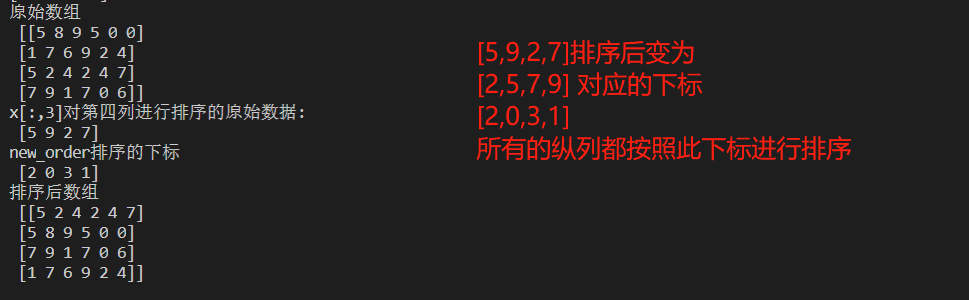# python中的排序

import numpy as np
#排序中主要用到的方法是 np.sort   np.argsort

x = np.array ([2,1,4,3,5])
print(np.sort(x))
#argsort返回的是排好序的数组的原始index
print(np.argsort(x))

# 沿着行或列进行排序
np.random.seed(1)
x = np.random.randint(0,10,(4,6))
print("x\n",x)
print("--->\n",x[:,2])
# print("纵向排序\n",np.sort(x,axis=0))
# print("横向排序\n",np.sort(x,axis=1))

#如果想要按照某一维度来进行排序 argsort
#通过这个组合，就完成了按照某一维度进行排序的方式
new_order = np.argsort(x[:,3])
print("x[:,3]:",x[:,3])
print("new_order\n",np.argsort(x[:,3]))
#所有的列都按照第四列的方式排序 原有数据 按照最新的下标[2,0,3,1]排列
#也就是原来第三个元素排在第一位 原来第一的元素 排在第二位
print("zzzz\n",x[new_order])01-261万+10-314588
03-176688
04-262万+
10-145649
04-133310
03-071万+
07-03164
04-28971
10-31241
06-0654
08-20
01-241万+